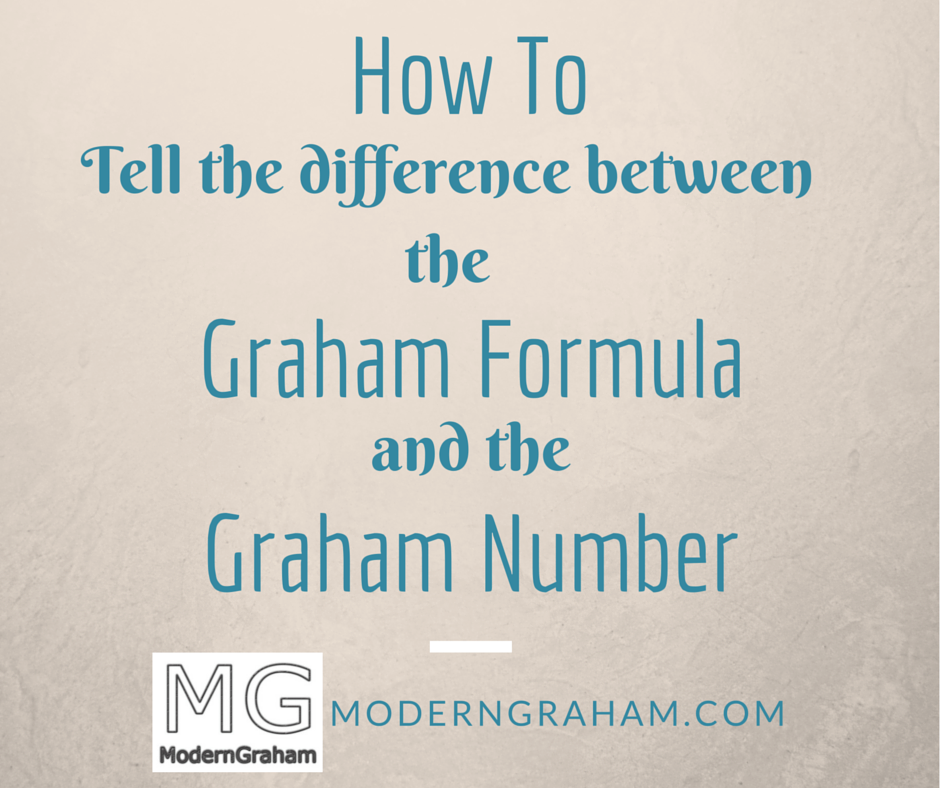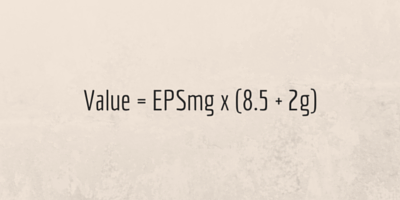# How to Tell the Difference Between the Graham Formula and the Graham Number## Get The Full Warren Buffett Series in PDF

Get the entire 10-part series on Warren Buffett in PDF. Save it to your desktop, read it on your tablet, or email to your colleagues

Often I get questions about the Graham Formula versus the Graham Number. There seems to be some misunderstanding out there between the two concepts, and this article will aim to help you as a reader understand the difference between the Graham Formula and the Graham Number. Both figures can be useful in their own ways, and can be combined in the ModernGraham approach.

# What is the Graham Formula?

The Graham Formula is the formula which Benjamin Graham provided in his classic book The Intelligent Investor. Specifically, the formula Graham recommended is:According to Graham, this formula resulted from a study of various valuation methods and is to be considered an effective shorthand way of estimating the intrinsic value of stocks. The formula should result in figures “fairly close to those resulting from the more refined mathematical calculations.”

# Where does the Graham Formula Come From?

Graham placed the Graham Formula in Chapter 11 of The Intelligent Investor, titled “Security Analysis for the Lay Investor: General Approach.” This chapter deals specifically with finding ways to simplify some of the complex mathematical analysis methods which have grown in popularity over the years. After dealing with analyzing bonds and preferred stocks, Graham addresses the issue of valuing stocks, beginning with the sentence “The ideal form of common-stock analysis leads to a valuation of the issue which can be compared with the current price to determine whether or not the security is an attractive purchase.”

Graham then went into some detail regarding how many valuation methods utilize future estimates of various figures such as sales, operating margin, etc. in order to then capitalize those figures back to the present in order to determine a value based on the future earnings of the company. However, he then said that “The reader will note that quite a number of the individual forecasts were wide of the mark.” Further, the present value of future earnings is widely dependent on various factors such as:

1. General Long-Term Prospects.
2. Management.
3. Financial Strength and Capital Structure.
4. Dividend Record.
5. Current Dividend Rate.

Discussion of each of those factors is beyond the intended scope of this particular article, but together those items constitute items that an analyst must consider when determining an intrinsic value.

With all of that in mind, it makes sense that Graham then proceeded to provide the Graham Formula as a way to simplify some of the process for the lay investor. Analysts can spend a lot of time considering each and every little detail about what they think a company is going to do, generating complex mathematical formulas and scenarios in order to determine a value, but Graham's formula is intended to help the average Intelligent Investor to estimate a value while making as few assumptions about the company as possible.

Immediately after listing the formula itself, Graham stated that “The growth figure should be that expected over the next seven to ten years.” He also included a footnote on that sentence. The footnote reads:

Note that we do not suggest that this formula gives the “true value” of a growth stock, but only that it approximates the results of the more elaborate calculations in vogue.”

Some have taken that footnote to mean that the Graham Formula should not be used for estimating an intrinsic value, but I consider the footnote to be more of a reminder from Graham that the calculation of an intrinsic value is not an exact science and cannot be done with 100% certainty. Rather, the investor can only estimate at the intrinsic value due to the vast number of variables involved. Graham provided this formula specifically as a way to approximate the more complex formulas used in an analysis in order to give a “foreshortened and quite simple formula for the valuation of growth stocks, which is intended to produce figures fairly close to those resulting from the more refined mathematical calculations.”

Therefore, the Graham Formula is to be used for estimating intrinsic value within a margin of safety which will accommodate the possibility of error in calculation.

# What is the Graham Number?

The Graham Number is a figure used by some investors as an upper limit to how much an investor should pay for a stock. Here's an example of how some investors use the Graham Number when analyzing dividend aristocrats. The Graham Number is calculated using this formula:According to the Graham Number calculation, the price must be below the square root of the product of 22.5, the Earnings Per Share, and the Book Value Per Share.

# Where does the Graham Number Come From?

The Graham Number formula was never actually provided by Benjamin Graham. Rather, it seems to be engineered out of one of Graham's recommended requirements for the Defensive Investor. In Chapter 14 of The Intelligent Investor, Graham provided a list of suggested criteria to help the Defensive Investor find quality securities for consideration. Those criteria are as follows:

1. Adequate Size of the Enterprise
2. A Sufficiently Strong Financial Condition
3. Earnings Stability
4. Dividend Record
5. Earnings Growth
6. Moderate Price / Earnings Ratio
7. Moderate Ratio of Price to Assets

In the seventh criteria, Moderate Ratio of Price to Assets, Graham says that “Current prices should not be more than 1.5 times the book value last reported. However, a multiplier of earnings below 15 could justify a correspondingly higher multiple of assets. As a rule of thumb we suggest that the product of the multiplier times the ratio of price to book value should not exceed 22.5.” The 22.5 number comes from the product of his suggested maximum price to earnings ratio of 15 and the suggested maximum book value of 1.5.

Somewhere along the line, analysts took this suggestion from Graham and extrapolated it into the Graham Number.

# The Graham Number is Only a Shorthand Version of the Graham Requirements for Defensive Investors.

It is my belief that the Graham Number is only a way to easily and quickly screen companies to be used by individual investors not interested in applying all of Graham's suggested investment techniques. In some ways it seems to be a figure created to simplify Graham's work into a single recommendation, which to me seems to miss the overall point Graham is trying to make altogether.

The Defensive Investor requirements are intended to assist the investor in narrowing down his potential list of investments to only those that are of the highest quality. Graham provided a list of suggested requirements to achieve that purpose, while he specifically provided the Graham Formula as a metric for estimating the intrinsic value of companies. Why would Graham have listed the suggested criteria in an area separately from the formula if he did not intend both items to be used?

# What Approach Does ModernGraham Use?

Here on ModernGraham, I've developed our approach to utilize the full breadth of Graham's recommendations. This is through utilizing both the suggested selection criteria to narrow down the list of potential investments and through the use of the formula to estimate an intrinsic value. Doing this allows the investor to narrow down the focus to a select number of companies and then generate an estimated intrinsic value based on the Graham Formula for comparison to the current price.

Each ModernGraham valuation of a company begins with determining whether it is suitable for either the Defensive Investor or the Enterprising Investor, based on a modernized version of Benjamin Graham's suggested selection criteria for each investor type. Here's a great post on how you can determine which type of investor you are. After that step is completed, the valuation continues to determining an estimated intrinsic value for the company based on the ModernGraham formula.

The ModernGraham formula has been modified slightly from the Graham Formula, only in the sense that it uses a weighted-average of five years of earnings data (EPSmg). The rationale is that Graham suggested using a normalized earnings per share figure in order to smooth out the effects of the business cycle, and also specifically suggested taking an average of earnings per share data when using the figure in analysis. You can learn more about how to estimate a growth rate in this post. Here's the ModernGraham Formula:This formula is specifically intended to provide only an estimate of the company's intrinsic value and must be utilized in tandem with a margin of safety because it does not provide an exact figure but only approximates some of the more complicated valuation methods.

ModernGraham utilizes multiple layers of safety margins including:

1. Maximum possible estimated growth rate of 15%
2. Estimated growth rate is reduced by 25%
3. To receive a rating of “undervalued” a company must be trading at 75% or less of its intrinsic value.
4. To receive a rating of “overvalued” a company must be trading at 110% or more of its intrinsic value.

Each valuation is intended to be a useful source for investors to utilize when conducting research into investment opportunities, and after the first two steps of the ModernGraham analysis, investors are encouraged to continue their research in order to determine if the opportunity is right for their own individual situation.

# Where Can You Find the Graham Number?

Even though the Graham Number isn't used in the ModernGraham approach, the figure is now provided with each individual ModernGraham valuation. In addition, as of today all new valuations will include a chart showing the Graham Number over time in comparison to the stock price. For those investors who place an emphasis on utilizing the Graham Number, this can be a great tool to see how the strategy has performed over time with respect to the specific company.

Be sure to become a premium member today to gain access to all of the individual ModernGraham valuations!